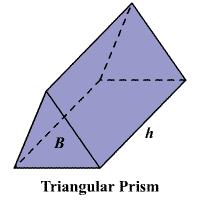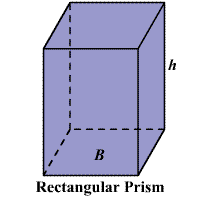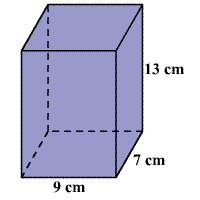# Volume of a Prism

A prism is a polyhedron with two parallel, congruent faces called bases that are polygons .The volume of a $3$ -dimensional solid is the amount of space it occupies.  Volume is measured in cubic units ( ${\text{in}}^{3},{\text{ft}}^{3},{\text{cm}}^{3},{\text{m}}^{3}$ , et cetera).  Be sure that all of the measurements are in the same unit before computing the volume.

The volume $V$ of a prism is the area of the base $B$ times the height $h$ .

$V=Bh$

Note: A cubic centimeter ( ${\text{cm}}^{3}$ ) is a cube whose edges measure $1$ centimeter.

Example:

Find the volume of the prism shown.Solution

The formula for the volume of a prism is $V=Bh$ , where $B$ is the base area and $h$ is the height.

The base of the prism is a rectangle. The length of the rectangle is $9$ cm and the width is $7$ cm.

The area $A$ of a rectangle with length $l$ and width $w$ is $A=lw$ .

So, the base area is $9×7$ or $63\text{\hspace{0.17em}}\text{\hspace{0.17em}}{\text{cm}}^{2}$ .

The height of the prism is $13$ cm.

Substitute $63$ for $B$ and $13$ for $h$ in $V=Bh$ .

$V=\left(63\right)\left(13\right)$

Multiply.

$V=819$

Therefore, the volume of the prism is $819$ cubic centimeters.